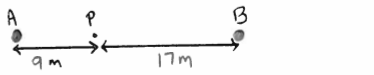Problem: Antennas A and B emit coherent radio waves that have wavelength 8.0 m and that are in phase. Point P is on the line connecting the two antennas and is 9.0 m from A and 17.0 m from B. Is the interference at point P for the waves from the two antennas constructive or destructive?(a) destructive(b) constructive

FREE Expert Solution
79% (393 ratings)
Problem Details

Antennas A and B emit coherent radio waves that have wavelength 8.0 m and that are in phase. Point P is on the line connecting the two antennas and is 9.0 m from A and 17.0 m from B. Is the interference at point P for the waves from the two antennas constructive or destructive?

(a) destructive

(b) constructive